CAT  >  Practice Questions Level 2: Exponents & Logarithm - 1

# Practice Questions Level 2: Exponents & Logarithm - 1 - Notes | Study Level-wise Practice Questions for CAT Preparation - CAT

 1 Crore+ students have signed up on EduRev. Have you?

This EduRev document offers 10 Multiple Choice Questions (MCQs) from the topic Exponents & Logarithm (Level - 2). These questions are of Level - 2 difficulty and will assist you in the preparation of CAT & other MBA exams. You can practice/attempt these CAT Multiple Choice Questions (MCQs) and check the explanations for a better understanding of the topic.

Question for Practice Questions Level 2: Exponents & Logarithm - 1
Try yourself:If loga p = 2.3, the value of 3 loga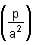is

Question for Practice Questions Level 2: Exponents & Logarithm - 1
Try yourself:If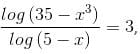then the value of x could be

Question for Practice Questions Level 2: Exponents & Logarithm - 1
Try yourself:If log x3 + log 6a = log 162 + log a, find the value of x.

Question for Practice Questions Level 2: Exponents & Logarithm - 1
Try yourself:If x3/7 = 423, the value of log x3 is

Question for Practice Questions Level 2: Exponents & Logarithm - 1
Try yourself:Find the solution of the equation log7 log5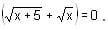Question for Practice Questions Level 2: Exponents & Logarithm - 1
Try yourself:What will you get after simplifying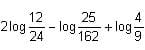?

Question for Practice Questions Level 2: Exponents & Logarithm - 1
Try yourself:The least value of the expression 2 log10 (x) - logx (0.01), for x > 1 is

Question for Practice Questions Level 2: Exponents & Logarithm - 1
Try yourself:Find the value of log10(tan 1°) + log10(tan 2°) + … + log10(tan 89°).

Question for Practice Questions Level 2: Exponents & Logarithm - 1
Try yourself:If f(n) = log2 3 × log3 4 × log4 5 ….. logn – 1 n, then the value of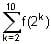is

Question for Practice Questions Level 2: Exponents & Logarithm - 1
Try yourself:The value of log2 3.log3 4.log4 5........ log98 99.log99 100 is

The document Practice Questions Level 2: Exponents & Logarithm - 1 - Notes | Study Level-wise Practice Questions for CAT Preparation - CAT is a part of the CAT Course Level-wise Practice Questions for CAT Preparation.
All you need of CAT at this link: CAT

## Level-wise Practice Questions for CAT Preparation

277 docs
 Use Code STAYHOME200 and get INR 200 additional OFF

## Level-wise Practice Questions for CAT Preparation

277 docs

### How to Prepare for CAT

Read our guide to prepare for CAT which is created by Toppers & the best Teachers

Track your progress, build streaks, highlight & save important lessons and more!

,

,

,

,

,

,

,

,

,

,

,

,

,

,

,

,

,

,

,

,

,

;